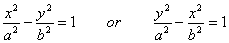# Equation of Hyperbola - Graphing Problems

Problems with detailed solutions on the hyperbola equation are presented in this tutorial.

## Review

A hyperbola with center at the origin (0,0), is the graph ofThe graph of the equation on the left has the following properties: x intercepts at ~+mn~ a , no y intercepts, foci at (-c , 0) and (c , 0), asymptotes with equations y = ~+mn~ x (b/a). The graph of the equation on the right has the following properties:  y intercepts at ~+mn~ a , no x intercepts, foci at (0 , -c) and (0 , c), asymptotes with equations y = ~+mn~ x (a/b) .

a, b and c are related by  c2 = a2 + b2.

The length of the transverse axis is 2a, and the length of the conjugate axis is 2b.

Applying the symmetry tests for graphs of equations in two variables, the hyperbola is symmetric with respect to x axis, y axis and the origin.

A tutorial on the definition and properties of hyperbolas can be found in this site.

### Problem 1

Given the following equation

9x2 - 16y2 = 144

a) Find the x and y intercepts, if possible,  of the graph of the equation.

b) Find the coordinates of the foci.

c) Sketch the graph of the equation.

Solution to Problem1

a) We first write the given equation in standard form by dividing both sides of the equation by 144

9x2 / 144  -  16y2 / 144  =  1

x2 / 16  -  y2 / 9  =  1

x2 / 42  -  y2 / 32  =  1

We now compare the equation obtained with the standard equation (left) in the review above and we can say that the given equation is that of an hyperbola with a = 4 and b = 3.

Set y = 0 in the equation obtained and find the x intercepts.

x2 / 42  = 1

Solve for x.

x2  = 42

x = ~+mn~ 4

Set x = 0 in the equation obtained and find the y intercepts.

- y2 / 32 = 1

NO y intercepts since the above equation does not have real solutions.

b) We need to find c first.

c2 = a2 + b2

a and b were found in part a).

c2 = 42 + 32

c2 = 25

Solve for c.

c = ~+mn~ 5

The foci are    F1 (5 , 0) and  F2 (-5 , 0)

c)

1 - Find the asymptotes y = - (b/a) x and y = (b/a) x and plot them.

y = -(3/4) x and y = (3/4) x

2 - plot the x intercepts

3 - Find extra points (if necessary)

set x = 6 and find y         9(6)2 - 16y2 = 144

- 16y2 = 144 - 324

y2 = 45 / 4

Solve for y y = 3(5)1/2 / 2 and y = - 3(5)1/2 / 2

so the points (6,  3(5)1/2 / 2) and    (6,  -3(5)1/2 / 2) are on the graph of the hyperbola.

Also because of the symmetry of the graph of the hyperbola, the points (-6,  3(5)1/2 / 2) and

(-6,  -3(5)1/2 / 2) are also on the graph of the hyperbola.Matched Problem:  Given the following equation

x2 - y2 = 9

a) Find the x and y intercepts, if possible,  of the graph of the equation.

b) Find the coordinates of the foci.

c) Sketch the graph of the equation.

## More References and Links to Hyperbolas

hyperbola equation
Find the Points of Intersection of Two Hyperbolas
Points of Intersection of a Hyperbola and a Line